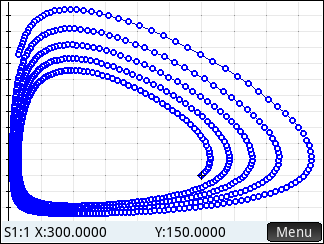Rabbit vs. Foxes
02-08-2020, 02:26 PM
Post: #1Eddie W. ShoreSenior Member Posts: 1,122 Joined: Dec 2013
Rabbit vs. Foxes
The program presented today is based on the Rabbits vs. Foxes program for the HP 25 (see source below). The program determines the population of rabbits and foxes over time as modeled by the differential equations:

Change in Rabbits:
dr/dt = 2 * r - α * r * f

Change of Foxes:
df/dt = -f + α * r * f

where:
r = population of rabbits
f = population of foxes
α = probability of a rabbit encounters a fox
h = step

This is approximated by Euler's method.

The HP Prime program RABBIT25 displays a print screen of results of the desired amount of iterations. The HP 42S program RAB25 displays the results one step at time in the format rrrrr.fffff (rabbits.foxes), like the original HP 25 program.

HP Prime Program RABBIT25
Code:
 EXPORT RABBIT25() BEGIN // 2020-01-20 EWS // Based on the Rabbits vs // Foxes HP 25 program LOCAL α,h,r,f,k,n,a; // initialize and input INPUT({α,h,r,f,n}, "Rabbits vs Foxes", {"α: ","h: ","r0:","f0:","n: "}, {"α","h","inital # rabbits", "initial # foxes", "# iterations"}); L0:={r}; L1:={f}; // compute data MSGBOX("L0 = rabbit population, L1 = fox population; (0,1,2,...,n), size n+1"); HFormat:=0; PRINT(); PRINT("Rabbits vs Foxes"); FOR k FROM 0 TO n DO IF k≠0 THEN a:=α*r*f; r:=r+h*(2*r-a); f:=f+h*(−f+a); END; PRINT(k+" R: "+IP(r)+" F: "+ IP(f)); END; // end of program END;

Source:

Randall B. Neff and Lynn Tilman "An Example of HP-25 Programming" Hewlett-Packard Journal: November 1975. pg. 6

Blog Post: http://edspi31415.blogspot.com/2020/02/h...foxes.html
02-08-2020, 07:52 PM
Post: #2Mark PowerMember Posts: 59 Joined: Dec 2013
RE: Rabbit vs. Foxes
Here is another variation on this program from Datafile V36N3P27 as presented at the HPCC Mini-Conference in October 2019. This version dispenses with the dialogue box entry of values, but adds saving the results in a matrix and hence plotting and exploring the results in the graphing environment.The Prime requires that you have opened the Statistics 2Var before entering the program, otherwise a syntax error is generated.

The complete graph of results (1125 iterations), as shown in the original HP magazine article, is drawn in approximately 0.33s on an HP Prime G2.

PHP Code:
EXPORT Rabbits_vs_Foxes()BEGIN LOCAL α:=0.01; LOCAL h:=0.02; LOCAL r0:=300; LOCAL f0:=150; LOCAL iterations:=1125; LOCAL n; LOCAL r:=MAKELIST(0,X,1,iterations); LOCAL f:=MAKELIST(0,X,1,iterations); r:=r0; f:=f0; FOR n FROM 1 to iterations-1 DO  r[n+1]:=r[n]+h*(2*r[n]-α*r[n]*f[n]);  f[n+1]:=f[n]+h*(-f[n]+α*r[n]*f[n]);  END; STARTAPP("Statistics 2Var"); C1:=r; C2:=f; SetIndep(S1,C1); SetDepend(S1,C2); Connect:=1; Fit:=0; Xtick:=50; Ytick:=50; STARTVIEW(9);END;
 « Next Oldest | Next Newest »

User(s) browsing this thread: 1 Guest(s)